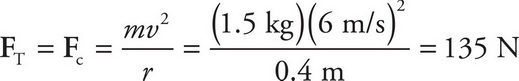# AP Physics 1 Question 399: Answer and Explanation

### Test Information

Question: 399

7. A student attaches a 1.5 kg mass to a 40 cm long string. The student then begins to whirl the mass in a horizontal circle with increasing speed. If the string snaps when the mass has a speed of 6 m/s, what is the breaking strength of the string?

• A. 22.5 N
• B. 135 N
• C. 240 N
• D. 360 N